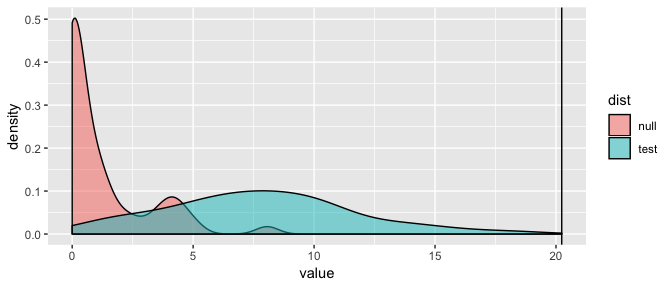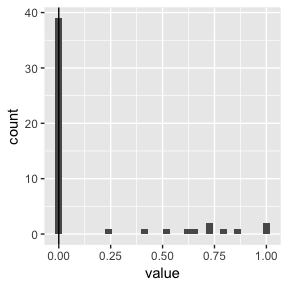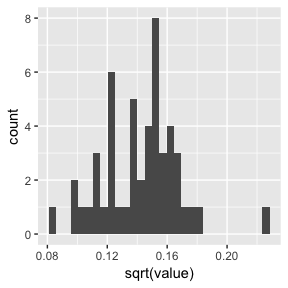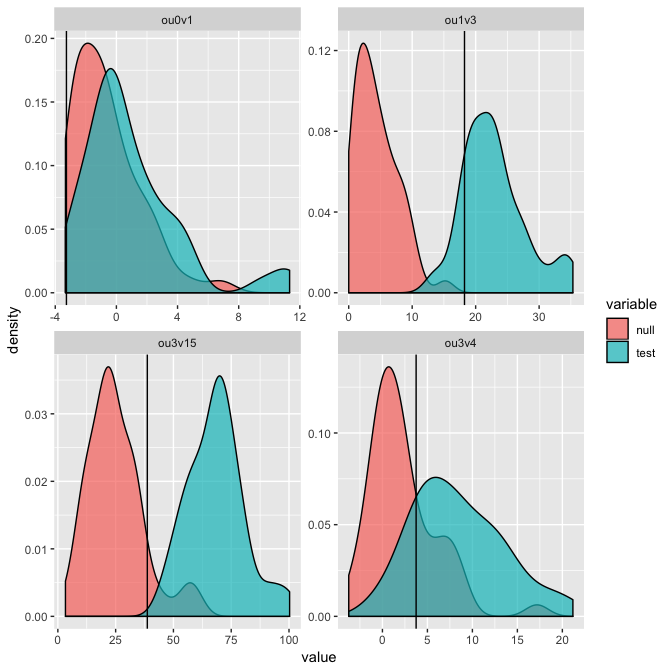# pmc tutorial

#### 2018-05-17

Beta, use with caution!

This is a lightweight implementation of my pmc package focusing on what I think are the more common use cases (e.g. it will no longer support comparisons of a geiger model against an ouch model). Further, it does not cover many of the newer model fitting that have been implemented since pmc was first released.

The goal of this release is mostly to provide compatibility with current versions of geiger.

## Getting started

knitr::opts_chunk$set(comment=NA, warning=FALSE, message=FALSE) Install the package: library("devtools") install_github("cboettig/pmc2") library("pmc") library("geiger") library("ouch") library("ggplot2") library("tidyr") library("dplyr") library("gridExtra") A trivial example with data simulated from the lambda model. cores <- 1 # parallel cores available nboot <- 50 # too small, but vignette gotta build faster phy <- sim.bdtree(n=10) dat <- sim.char(rescale(phy, "lambda", .5), 1)[,1,] out <- pmc(phy, dat, "BM", "lambda", nboot = nboot, mc.cores = cores) Plot the results: dists <- data.frame(null = out$null, test = out$test) dists %>% gather(dist, value) %>% ggplot(aes(value, fill = dist)) + geom_density(alpha = 0.5) + geom_vline(xintercept = out$lr)## Finches Examples

The first set of examples uses the finches data and geiger functions~ to look at uncertainty in parameter estimates using the pmc method. We start off by loading the required libraries

data(geospiza)
bm_v_lambda <- pmc(geospiza$phy, geospiza$dat[, "wingL"],
"BM", "lambda", nboot = nboot, mc.cores = cores) 

Currently the output will only run for a single trait at a time, for efficient memory usage. Here we specify the wing length trait.

We can analyze the parameter distributions as presented in the manuscript.
For instance, we can look at a histogram of values of lambda obtained from the different simulations. Because the pmc approach runs four different fits:

there are actually 4 different parameter distributions we can use.
The comparisons AA'' andBB’’ are the typical way one would bootstrap the model fits. All of these combinations are returned in the data set which is one of the items in the list returned by pmc (which we have named above).
Subsetting is a good way to get the parameter of interest, lambda, for the comparison of interest, BB. (Note that for comparisons AA and AB, which fit model Brownian motion, there is of course no parameter lambda).

lambdas <- bm_v_lambda$par_dists %>% filter(comparison=="BB", parameter=="lambda")  The returned list from pmc also stores the two models it fit to the original data, inder the names A and B. We can use this to extract the value of lambda estimated on model B from the raw data: est <- coef(bm_v_lambda[["B"]])[["lambda"]] ggplot(lambdas) + geom_histogram(aes(value)) + geom_vline(xintercept=est)Note that the ability to estimate lambda is very poor, with most simulations returning an estimate of almost zero despite the true value used in the simulations being . Estimating the sigma parameter is somewhat more reliable, even on this small tree: bm_v_lambda$par_dists %>% filter(comparison=="BB", parameter=="sigsq") %>%
ggplot() + geom_histogram(aes(sqrt(value)))## Anoles example

Next we consider the examples re-analyzing the Anoles data from @Butler2004, using methods from the ouch package.

data(anoles)
tree <- with(anoles, ouchtree(node, ancestor, time / max(time), species))

ou3v4 <- pmc(tree, log(anoles["size"]), modelA = "hansen", modelB = "hansen",
optionsA = list(regimes = anoles["OU.LP"]),
optionsB = list(regimes = anoles["OU.4"]),
nboot = nboot, sqrt.alpha = 1, sigma = 1, mc.cores = cores)

ou3v15 <- pmc(tree, log(anoles["size"]), "hansen", "hansen",
list(regimes = anoles["OU.LP"]),
list(regimes = anoles["OU.15"]),
nboot = nboot, sqrt.alpha = 1, sigma = 1, mc.cores = cores)

ou1v3 <- pmc(tree, log(anoles["size"]), "hansen", "hansen",
list(regimes = anoles["OU.1"]),
list(regimes = anoles["OU.LP"]),
nboot = nboot, sqrt.alpha = 1, sigma = 1, mc.cores = cores)

ou0v1 <- pmc(tree, log(anoles["size"]), "brown", "hansen",
list(),
list(regimes = anoles["OU.1"], sqrt.alpha = 1, sigma = 1),
nboot = nboot, mc.cores = cores)
results <- bind_rows(
data.frame(comparison = "ou3v4", null = ou3v4$null, test = ou3v4$test, lr = ou3v4$lr), data.frame(comparison = "ou3v15", null = ou3v15$null, test = ou3v15$test, lr = ou3v15$lr),
data.frame(comparison = "ou1v3", null = ou1v3$null, test = ou1v3$test, lr = ou1v3$lr), data.frame(comparison = "ou0v1", null = ou0v1$null, test = ou0v1$test, lr = ou0v1$lr)) %>%
gather(variable, value, - comparison, - lr)
ggplot(results) +
geom_density(aes(value, fill = variable), alpha=0.7) +
geom_vline(aes(xintercept=lr)) +
facet_wrap(~ comparison, scales="free")## Citation

Carl Boettiger, Graham Coop, Peter Ralph (2012) Is your phylogeny informative? Measuring the power of comparative methods, Evolution 66 (7) 2240-51.